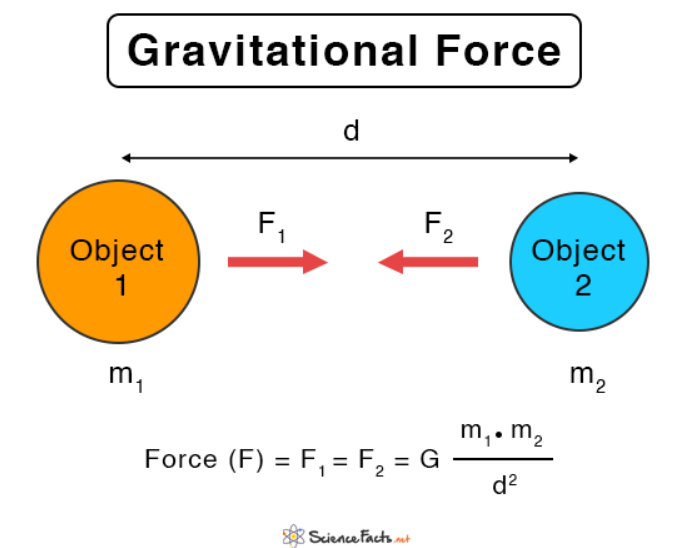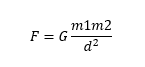Ravela Da Cruz

# Gravitational Force Study Guide

## Introduction

Ever heard of the phrase “what goes up must come down”? The idea has been understood for as long as humans have been around. Our prehistoric ancestors threw clubs and spears into the air to hunt, whereas we now play baseball and javelin throwing as a sport. Come to think of it, even the Moon is trying to fall into the earth, but it is traveling so fast that it keeps missing! 😃 The same applies to planets, and the sun.

Gravity constantly acts on these objects to bring them close to one another. In this chapter, we will learn about how this mysterious force keeps all things in place, so let’s get started!Source

### What is the Gravitational Force?

In simple terms, gravity is an attractive force that exists between all objects that have mass. Even the tiniest grains of sand have gravitational forces acting on them that attract the particles together. By definition, the gravitational force between two objects is the force of attraction directly proportional to their masses and inversely proportional to the square of the distance between them.

### Mathematical Expression for Gravitational ForceSource

From the definition above, we see there are two factors that affect the gravitational force between two objects:

• Mass of the objects
• Distance between the objects

Consider two objects having masses m1 and m2 spaced apart by a distance (d). By definition the gravitational force of attraction (F) between these objects is given by the relation :Here G, is the universal gravitational constant at 6.67 × 10-11 N·m2/kg2.

So why don’t apples and oranges on your dining table just roll over and stick together? It’s because the gravitational force of the earth on each fruit is stronger. While gravitational forces try to bring the apple and the orange together, the fruits are more strongly held in their place by the earth’s gravity.

Let’s say you take the same apple and orange out into space away from all planets, and stars; then they would easily attract and bump into each other!

Based on the formula above, we have two major takeaways regarding this 'attractive' force between two objects:

• Gravitational force is directly proportional to the mass of the objects.
• This also implies that an object of heavier mass will pull an object of smaller mass towards it - think of it as an intense game of tug of war!

Gravitational force is indirectly proportional to the distance between the objects.

### Gravitational Force fun facts

Gravity is key to the balance in our universe, and here are a few instances where gravity plays a huge role:

1. The gravitational force also determines your weight. Yep, you read that right! The acceleration produced by gravity which is a constant denoted as ‘g’ determines how much you weigh. The moon only has 1/6th of Earth’s gravity. If you weigh 40 kilograms on earth, your weight on the moon will be 6.6 kilograms! However the sun’s gravity is 27.9 times that of the earth, so if you were to stand on the sun (hypothetically) your weight would be about 1,116 kilograms!

2. Gravity is the weakest of the 4 fundamental forces. The other three are the electromagnetic force, the weak nuclear force, and the strong nuclear force.

3. A tiny magnet has enough electromagnetic force to overcome the Earth’s gravity and stick to your refrigerator’s door 😃

## Conclusion:

• A gravitational force can be described as a force of attraction between two or more objects.
• The gravitational force increases as the mass of the object increases.
• The gravitational force decreases as the distance between the objects increases.
• A gravitational force keeps everything in place on the earth.

### FAQs:

1. What is an example of gravitational force?

When you throw a ball into the air, the ball eventually stops traveling towards the sky and comes back down. This is because the earth's gravitational force pulls the ball back down.

2. What does 9.8 m S² mean?

The acceleration due to gravity (g) on the earth is 9.8 m/ S². It means that any object dropped from a height (neglecting air resistance) will accelerate at a rate of 9.8 m/ S². A ball dropped from a cliff will be falling at 9.8 m/s, one second after it is dropped. At 2 seconds, it will be falling at 19.6 m/s; at 3 seconds, it will be falling at 29.4 m/s, and so on.

We hope you enjoyed studying this lesson and learned something cool about Gravitational Force! Join our Discord community to get any questions you may have answered and to engage with other students just like you! Don't forget to download our App to experience our fun, VR classrooms - we promise, it makes studying much more fun! 😎

## Sources:

1. 5.3 Newton's Universal Law of Gravity. https://flexbooks.ck12.org/cbook/ck-12-physics-flexbook-2.0/section/5.3/primary/lesson/universal-law-of-gravity-phys/. Accessed 27 Jan 2022.
2. What does 9.8 N/kg mean?. https://byjus.com/jee-questions/what-does-nine-point-eight-n-kg-mean/#:~:text=9.8%20N%2Fkg%20is%20the,a%201%20kg%20of%20mass.&text=The%20gravitational%20strength%20on%20the,or%209.8%20m%2Fs2. Accessed 27 Jan 2022.
3. The Acceleration of Gravity. https://www.physicsclassroom.com/class/1DKin/Lesson-5/Acceleration-of-Gravity. Accessed 27 Jan 2022.